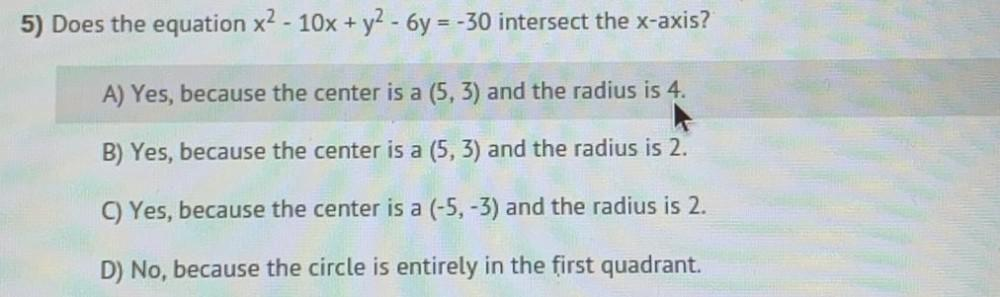Question:

# 5) Does the equation x² - 10x + y² - 6y = -30 intersect the x-axis? A) Yes, because the center is a (5, 3) and the radius is 4. B) Yes, because the center is a (5, 3) and the radius is 2. C) Yes, beca5) Does the equation x² - 10x + y² - 6y = -30 intersect the x-axis? A) Yes, because the center is a (5, 3) and the radius is 4. B) Yes, because the center is a (5, 3) and the radius is 2. C) Yes, because the center is a (-5, -3) and the radius is 2. D) No, because the circle is entirely in the first quadrant.